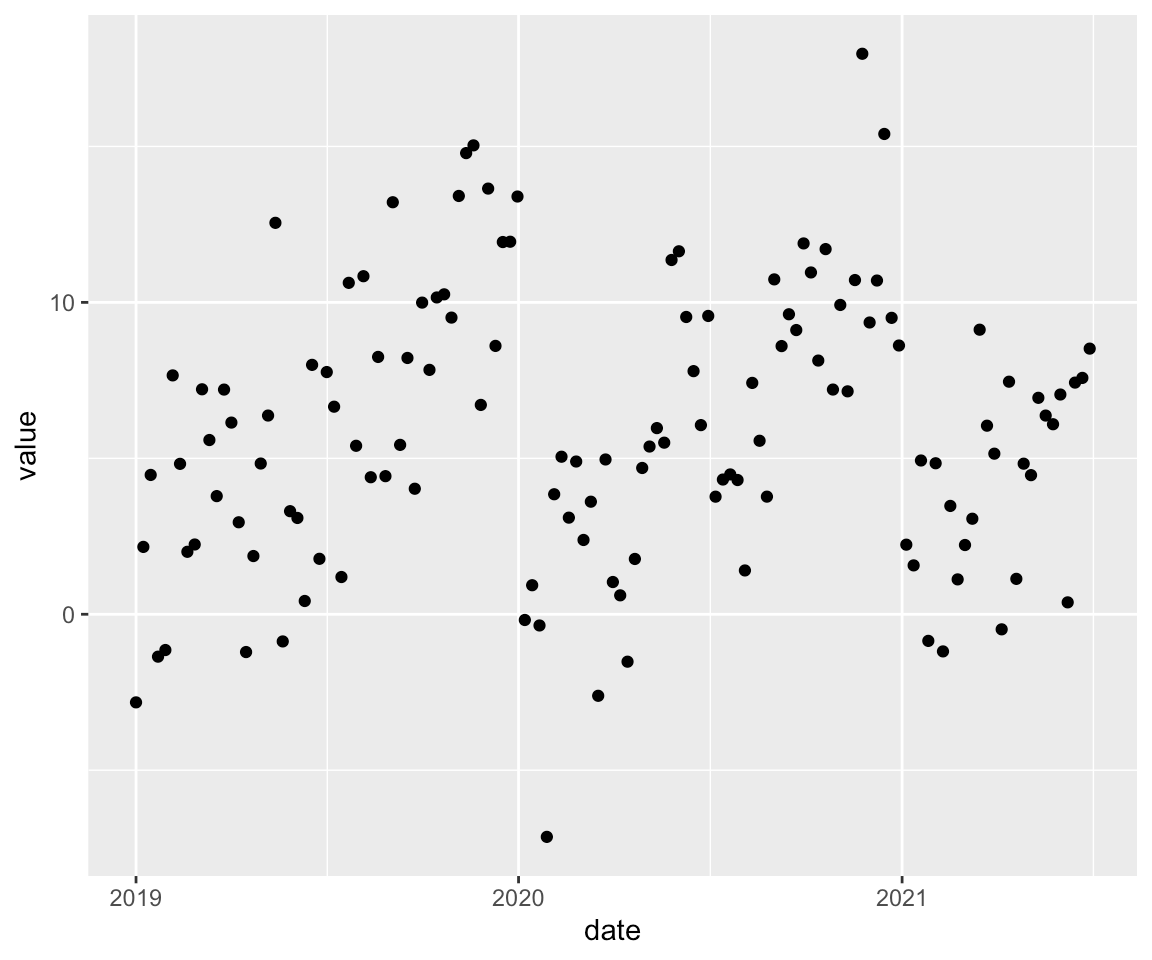Want to share your content on R-bloggers? click here if you have a blog, or here if you don't.

Sometimes you generate lots of ggplots of a similar kind, e.g. visualizations of different timeseries. Then you want to highlight some dates where something special had happened and you want to show that the value you are plotting changed at these dates.

So it would be nice if we can refactor these `geom_vline` or `geom_hline` calls into one single function.

Unfortunately the straight way using

 ```1 2 3 4 5 ``` ```add_lines <- function() { geom_vline(xintercept = ymd("2019-07-01"), color = "green") + geom_vline(xintercept = ymd("2021-01-01"), color = "red") + geom_hline(yintercept = c(0, 12), color = "blue") } ```

does not work:

 ``` 1 2 3 4 5 6 7 8 9 10 11 12 13 14 15 16 17 ``` ```library(ggplot2) library(dplyr, warn.conflicts = FALSE) library(lubridate, warn.conflicts = FALSE) data <- tibble( date = seq(from = ymd("2019-01-01"), to = ymd("2021-06-30"), by = "week"), ) %>% rowwise() %>% mutate( value = month(date) + rnorm(1, mean = 0, sd = 3) ) plot <- data %>% ggplot(aes(date, value)) + geom_point() plot ```Now we want to add some vertical and horizontal lines to `plot`:

 ```1 2 3 4 ``` ```plot + geom_vline(xintercept = ymd("2019-07-01"), color = "green") + geom_vline(xintercept = ymd("2021-01-01"), color = "red") + geom_hline(yintercept = c(0, 12), color = "blue") ```So let’s try it and use a function for adding these lines:

 ```1 ``` ```plot + add_lines() ```
 ```1 ``` ```## Error: Cannot add ggproto objects together. Did you forget to add this object to a ggplot object? ```

So we have to choose another way. The function should return instead of the sum of the function calls a list of these geom-calls!

 ```1 2 3 4 5 6 7 8 9 ``` ```add_lines_to_plot <- function() { list( geom_vline(xintercept = ymd("2019-07-01"), color = "green"), geom_vline(xintercept = ymd("2021-01-01"), color = "red"), geom_hline(yintercept = c(0, 12), color = "blue") ) } plot + add_lines_to_plot() ```Now it’s easy to reuse this function and add the same lines to other plots.